Latest Banking jobs   »   IBPS reasoning

# Reasoning Quiz For IBPS Clerk Prelims 2023 -18th August

Directions (1-3): Study the following information carefully and answer the questions accordingly.
Manisha starts walking in west direction from point C and walks 16m to reach point D. From point D, she walks 34m in north direction to reach point E. Then she walks 16m in west direction and reaches point F. From point F, she takes a right turn and walks 10m to reach point G.

Q1. Point G is in which direction with respect to point D?
(a) South
(b) North-East
(c) North-West
(d) West
(e) None of these

Q2. What is the shortest distance between points E and G?
(a) 12m
(b) 5√79m
(c) 2√89m
(d) 9m
(e) None of these

Q3. Point C is in which direction with respect to point F?
(a) South
(b) South-East
(c) North-West
(d) West
(e) None of these

Directions (4-6): Study the following information carefully and answer the questions accordingly.
Point O is 6m north of point S. Point G is 4m south of point K and 6m west of point F. Point S is 6m west of point X. Point K is 5m east of point O.

Q4. Point X is in which direction with respect to point O?
(a) North
(b) South-East
(c) North-West
(d) East
(e) Can’t be determined

Q5. Point K is in which direction with respect to point S?
(a) East
(b) West
(c) North-East
(d) South-West
(e) None of these

Q6. Point F is in which direction with respect to point X?
(a) South – East
(b) West
(c) North-East
(d) South-West
(e) None of these

Directions (7-9): Study the following information carefully and answer the questions accordingly.
Point C is 16m south of point D. Point G is 10m north of point H. Point G is 20m west of point F which is 24m north of point E. Point I is 28m south of point J. Point E is 40m east of point D. Point I is 48m west of point H.

Q7. Point E is in which direction with respect to point I?
(a) East
(b) South
(c) North-West
(d) South-East
(e) Can’t be determined

Q8. What is the shortest distance between the points D and H?
(a) 30m
(b) 3√129m
(c) 2√149m
(d) 35m
(e) None of these

Q9. Point G is in which direction with respect to point C?
(a) North-West
(b) West
(c) South-West
(d) North-East
(e) None of these

Directions (10): Study the following information carefully and answer the given question.
Point J is 15m south of point V. Point L is 10m north of point H which is 15m east of point J. Point G is 5m south of point T which is 20m east of point V.

Q10. What is the shortest distance between points L and G?
(a) 10m
(b) 15m
(c) 5m
(d) 12m
(e) None of these

Solutions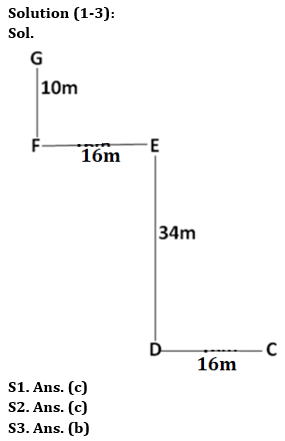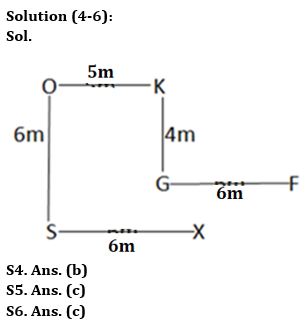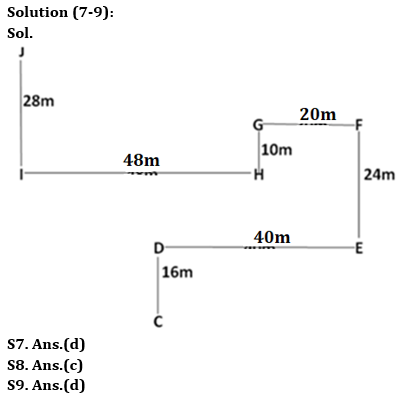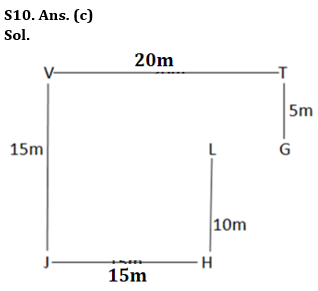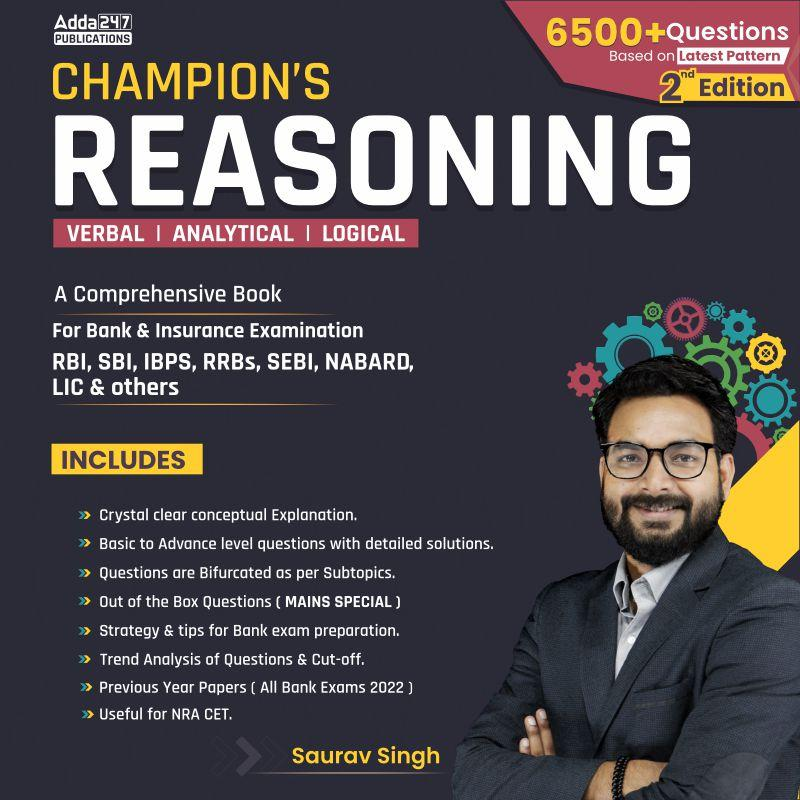## FAQs

### What is the selection process of IBPS Clerk 2023

The selection process of IBPS Clerk includes Prelims and Mains exam

#### Congratulations!Union Budget 2023-24: Free PDF